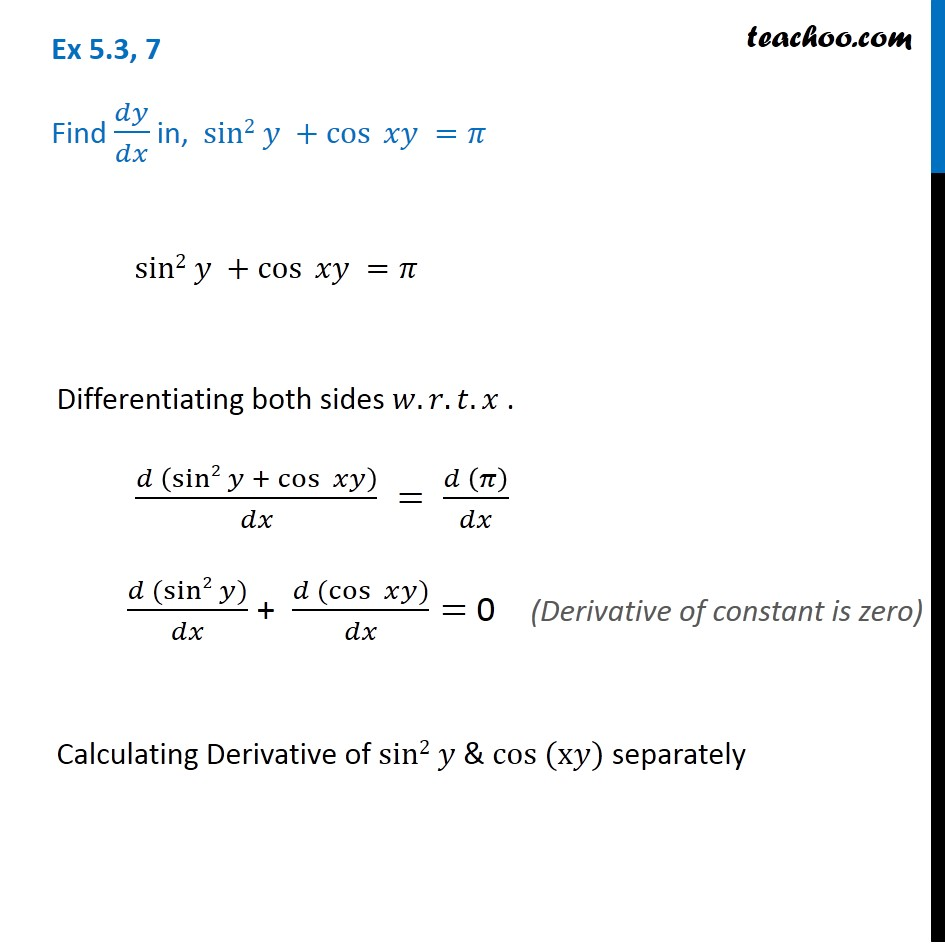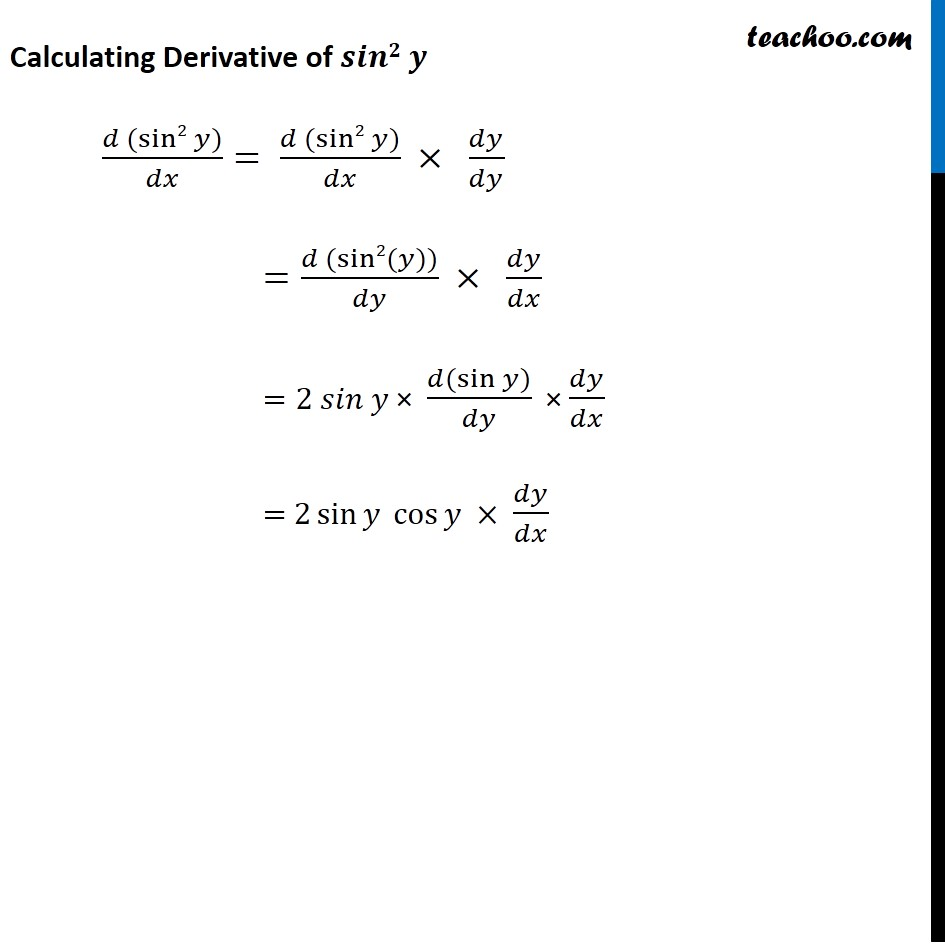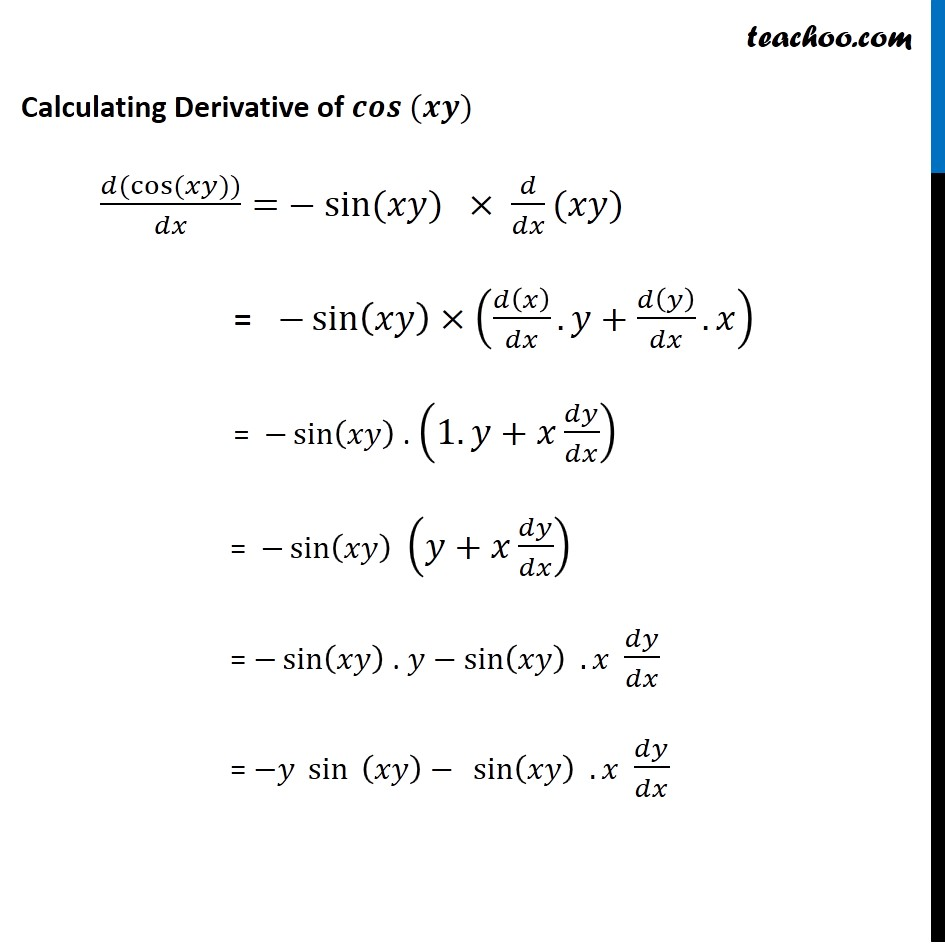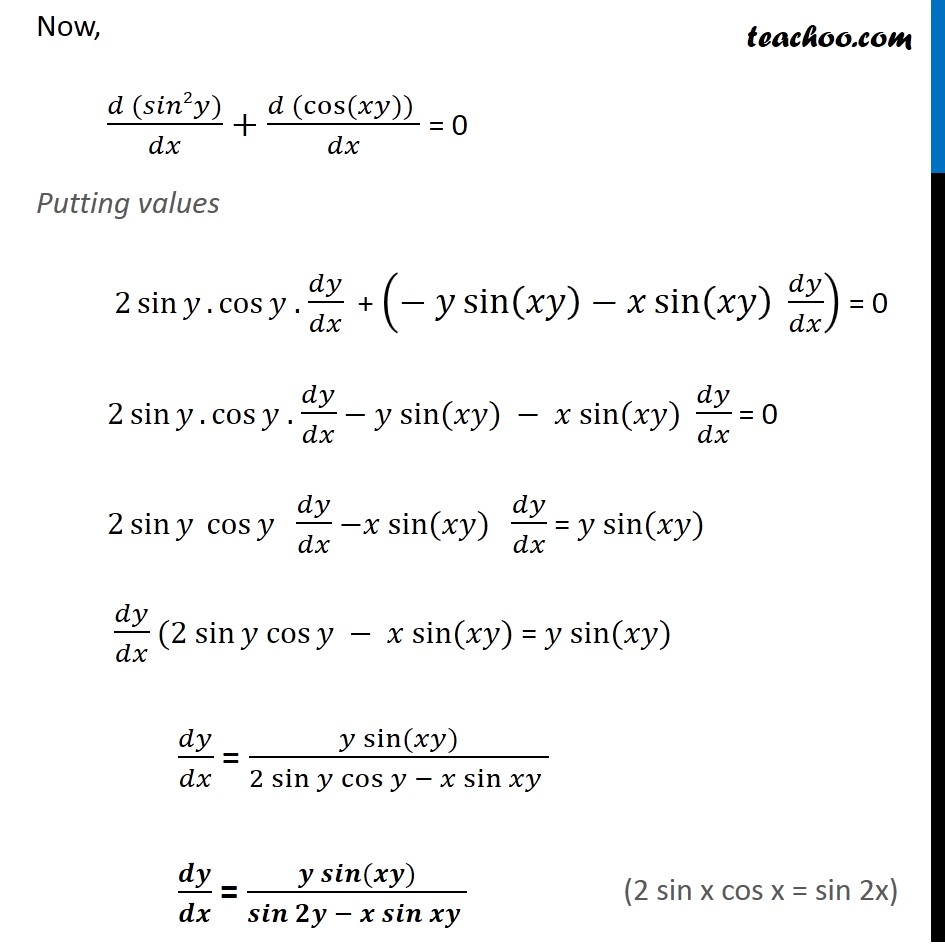Check Full Chapter Explained - Continuity and Differentiability - https://you.tube/Chapter-5-Class-12-Continuity1. Chapter 5 Class 12 Continuity and Differentiability
2. Serial order wise
3. Ex 5.3

Transcript

Ex 5.3, 7 Find 𝑑𝑦/𝑑𝑥 in, sin2 𝑦 +cos⁡ 𝑥𝑦 =𝜋 sin2 𝑦 +cos⁡ 𝑥𝑦 =𝜋 Differentiating both sides 𝑤.𝑟.𝑡.𝑥 . (𝑑 (sin2 𝑦 + cos⁡ 𝑥𝑦))/𝑑𝑥 = (𝑑 (𝜋))/𝑑𝑥 (𝑑 (sin2 𝑦))/𝑑𝑥 + (𝑑 (cos⁡〖 𝑥〗 𝑦))/𝑑𝑥= 0 Calculating Derivative of sin2 𝑦 & cos (x𝑦) separately (Derivative of constant is zero) Calculating Derivative of 𝒔𝒊𝒏𝟐 𝒚 (𝑑 (sin2 𝑦))/𝑑𝑥= (𝑑 (sin2 𝑦))/𝑑𝑥 × 𝑑𝑦/𝑑𝑦 =(𝑑 (sin2(𝑦)))/𝑑𝑦 × 𝑑𝑦/𝑑𝑥 =2 𝑠𝑖𝑛 𝑦 × (𝑑(sin⁡〖𝑦)〗)/𝑑𝑦 × 𝑑𝑦/𝑑𝑥 = 2 sin⁡〖𝑦 cos⁡〖𝑦 × 〗 〗 𝑑𝑦/𝑑𝑥 Calculating Derivative of 𝒄𝒐𝒔 (𝒙𝒚) (𝑑(cos⁡〖(𝑥𝑦))〗)/𝑑𝑥= − sin⁡(𝑥𝑦) × 𝑑/𝑑𝑥(𝑥𝑦) = −sin⁡(𝑥𝑦)×(𝑑(𝑥)/𝑑𝑥.𝑦+𝑑(𝑦)/𝑑𝑥.𝑥) = −sin⁡(𝑥𝑦) . (1.𝑦+𝑥 𝑑𝑦/𝑑𝑥) = −sin⁡(𝑥𝑦) (𝑦+𝑥 𝑑𝑦/𝑑𝑥) = −sin⁡(𝑥𝑦) . 𝑦−sin⁡〖(𝑥𝑦) . 𝑥〗 𝑑𝑦/𝑑𝑥 = −𝑦 sin⁡〖 (𝑥𝑦)〗 − sin⁡〖(𝑥𝑦) . 𝑥〗 𝑑𝑦/𝑑𝑥 Now, (𝑑 (𝑠𝑖𝑛2𝑦))/𝑑𝑥+(𝑑 (cos⁡〖(𝑥𝑦)) 〗)/𝑑𝑥 = 0 Putting values 2 sin⁡𝑦.cos⁡〖𝑦 .〗 𝑑𝑦/𝑑𝑥 + (− 𝑦 sin⁡(𝑥𝑦)−𝑥 sin⁡(𝑥𝑦) 𝑑𝑦/𝑑𝑥) = 0 2 sin⁡𝑦.cos⁡〖𝑦 .〗 𝑑𝑦/𝑑𝑥 − 𝑦 sin⁡(𝑥𝑦) − 𝑥 sin⁡(𝑥𝑦) 𝑑𝑦/𝑑𝑥 = 0 2 sin⁡𝑦 cos⁡〖𝑦 〗 𝑑𝑦/𝑑𝑥 −𝑥 sin⁡(𝑥𝑦) 𝑑𝑦/𝑑𝑥 = 𝑦 sin⁡(𝑥𝑦) 𝑑𝑦/𝑑𝑥 (2 sin⁡𝑦 cos⁡𝑦 − 𝑥 sin⁡(𝑥𝑦) = 𝑦 sin⁡(𝑥𝑦) 𝑑𝑦/𝑑𝑥 = (𝑦 sin⁡〖(𝑥𝑦)〗)/(2 〖sin 𝑦〗⁡〖cos⁡𝑦 − 𝑥 sin⁡𝑥𝑦 〗 ) 𝒅𝒚/𝒅𝒙 = (𝒚 𝒔𝒊𝒏⁡〖(𝒙𝒚)〗)/〖𝒔𝒊𝒏 𝟐𝒚〗⁡〖− 𝒙 𝒔𝒊𝒏⁡𝒙𝒚 〗 (2 sin x cos x = sin 2x)

Ex 5.3